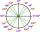# Cosine

The point (8, 6) is on the terminal side of angle θ.

cos θ =

« Correct result#### Solution:Leave us a comment of example and its solution (i.e. if it is still somewhat unclear...):Be the first to comment!#### To solve this example are needed these knowledge from mathematics:

See also our right triangle calculator. Most natural application of trigonometry and trigonometric functions is a calculation of the triangles. Common and less common calculations of different types of triangles offers our triangle calculator. Word trigonometry comes from Greek and literally means triangle calculation.

## Next similar examples:

1. FlowerbedFlowerbed has the shape of an isosceles obtuse triangle. Arm has a size 5.5 meters and an angle opposite to the base size is 94°. What is the distance from the base to opposite vertex?
2. CosineCalculate the cosine of the smallest internal angle in a right-angled triangle with cathetus 3 and 8 and with the hypotenuse 8.544.
3. LiftThe largest angle at which the lift rises is 16°31'. Give climb angle in permille.
4. Reference angleFind the reference angle of each angle:What is greater angle? -0.7 radians or -0.7π radians?
6. Theorem proveWe want to prove the sentence: If the natural number n is divisible by six, then n is divisible by three. From what assumption we started?
7. First manWhat is the likelihood of a random event where are five men and seven women first will leave the man?
8. TreesA certain species of tree grows an average of 0.5 cm per week. Write an equation for the sequence that represents the weekly height of this tree in centimeters if the measurements begin when the tree is 200 centimeters tall.
9. Nineteenth memberFind the nineteenth member of the arithmetic sequence: a1=33 d=5 find a19
10. 6 termsFind the first six terms of the sequence. a1 = 7, an = an-1 + 6
11. Volleyball8 girls wants to play volleyball against boys. On the field at one time can be six players per team. How many initial teams of this girls may trainer to choose?
12. One halfOne half of ? is: ?
13. Factory and divisionsThe factory consists of three auxiliary divisions total 2,406 employees. The second division has 76 employees less than 1st division and 3rd division has 212 employees more than the 2nd. How many employees has each division?
14. Linear systemSolve a set of two equations of two unknowns: 1.5x+1.2y=0.6 0.8x-0.2y=2
15. Two equationsSolve equations (use adding and subtracting of linear equations): -4x+11y=5 6x-11y=-5
16. PowersExpress the expression ? as the n-th power of the base 10.
17. Functions f,gFind g(1) if g(x) = 3x - x2 Find f(5) if f(x) = x + 1/2